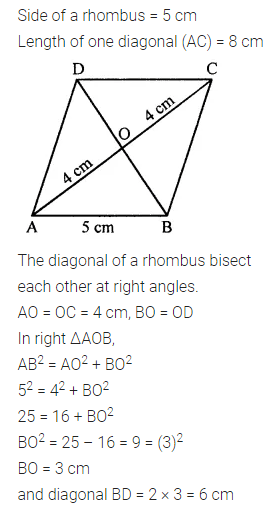# ML Aggarwal Class 7 Solutions for ICSE Maths Chapter 11 Triangles and its Properties Ex 11.5

## ML Aggarwal Class 7 Solutions for ICSE Maths Chapter 11 Triangles and its Properties Ex 11.5

Question 1.
PQR is a triangle, right-angled at P. If PQ = 10 cm and PR = 24 cm, find QR.
Solution: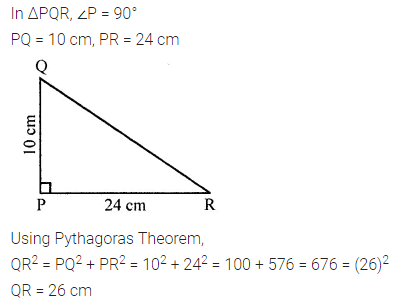Question 2.
ABC is a triangle, right-angled at C. If AB = 25 cm and AC = 7 cm, find BC.
Solution:Question 3.
Find the value of x in each of the following figures. All measurements are in centimeters.Solution: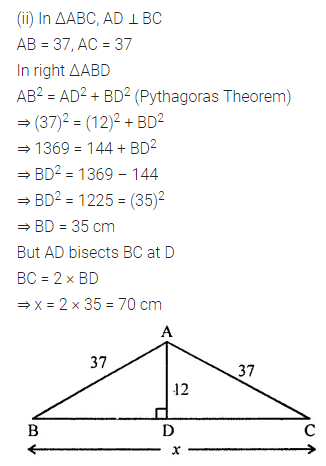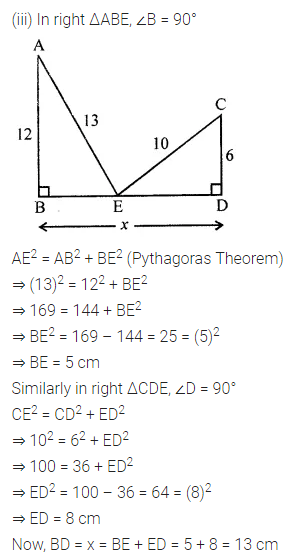Question 4.
Which of the following can be the sides of a right-angled triangle?
(i) 4 cm, 5 cm, 7 cm
(ii) 1.5 cm, 2 cm, 2.5 cm
(iii) 7 cm, 5.6 cm, 4.2 cm
Solution: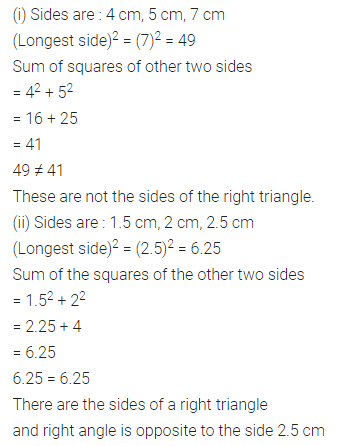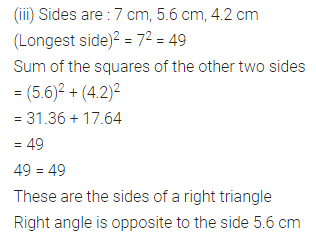Question 5.
A 15 m long ladder reaches a window 12 m high from the ground on placing it against a wall. How far is the foot of the ladder from the wall?
Solution:Question 6.
Find the area and the perimeter of the rectangle whose length is 15 cm and the length of one diagonal is 17 cm.
Solution: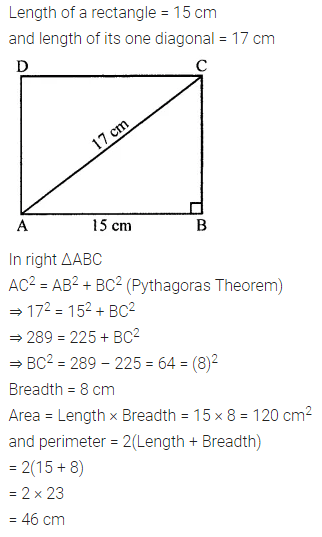Question 7.
If the diagonals of a rhombus measure 10 cm and 24 cm, find its perimeter.
Solution:Question 8.
The side of a rhombus is 5 cm. If the length of one diagonal of the rhombus is 8 cm, then find the length of the other diagonal.
Solution: